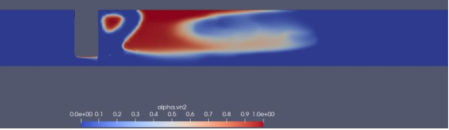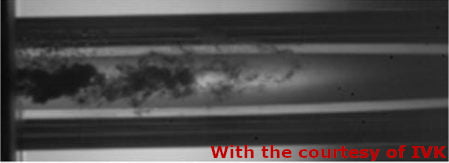# Multiphysics valve design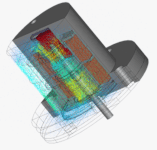## Structure

Using OOFELIE’s low-frequency electromagnetic (finite element) capabilities, engineers can use a multiphysics solver to compute the electromagnetc forces acting on the valve’s plunger (moving part) as a function of:

• the axial position of the mobile part
• the eccentricity
• the shape of the mobile part
• the current flowing in coils

Furthermore, 3D simulation results can be exported in table format for use in creating a reduced order model. This simplified model can then be exported and run in a system simulator. Tables can contain:

• Inductance as a function of the position and applied current.
• Force as a function of the position and applied current.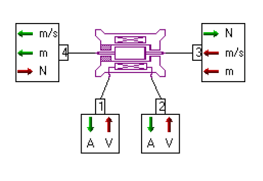## Cryogenic flow

Cryogenic flows are present in many industrial sectors (space, electronics, automotive, food, etc.). Accurate modelling of these flows is an essential step in the design of critical equipment such as valves or tanks.

Over the last few years, Open Engineering has acquired a solid experience in the calculation of cryogenic flows with phase change, particularly in the field of space valves.

Two types of calculation are proposed. The first calculation is based on an engineering model (also called “0D model”) which consists of a series of equations describing the physics of cavitation and flashing flows through an orifice. This model allows the type of regime (either sub-critical or critical) to be predicted very quickly and accurately as a function of the operating conditions. The following figure shows an example of a comparison between the model response and experimental results.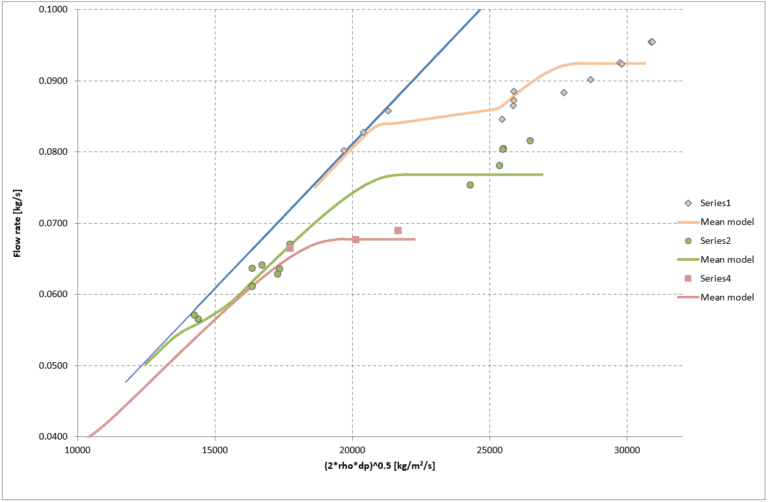The second calculation is based on a CFD solver coupled with a cavitation model. With this type of calculation, an accurate visualisation of the flow can be obtained, especially inside and downstream of the restriction.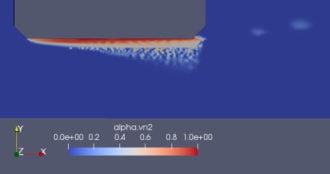The following figures show the calculated void fraction distributions; these are in very good agreement with the experimental visualisation.